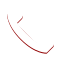Python 2020-04-15T06:25:57+00:00

# Python

Get familiar with Python using Data science & machine learning Techniques.

INR 40,000 + TaxesPython is an effective programming language that’s powerful, easy to learn and fast to code.
It is used for many different applications.

Over time, the huge community around this open source language has created a number of tools specifically for data science. As a result, analyzing data with Python has never been easier, and it is rapidly becoming ultimate choice for the Data scientists, when it comes to learning a computing language.

Just like other code of language, Python code can also be written like a traditional way, to execute an entire series of instructions at once, or it can also be executed line after line or block by block, making it perfect choice for working with data interactively.

## Course Overview

In this practical course you will get to learn all you need to get you started using Python for data analysis. Start with basic arithmetic and variables, and data structures, move on to how to load data from different sources, rearrange and aggregate it, and finally you will create your own stunning visualizations based on real data.

### Installation:

· Python Language Fundamentals

· Python Data Science Tools (Numpy)

· Panda’s Series & Data Frame

· Plotting & Visualization (matplotlib)

· Training under the guidance of experienced Faculty

· Classroom training with flexible timing

· Customized/On-demand training

· Provide Exclusive Study Materials

· Python via anaconda

· Jupyter Notebook

## CURRICULUM

#### Chapter 1: Python Language Fundamentals

Topic:1

• Variables, Data Types and Basic Syntax
• Operators and Expressions
• Control Flow & Loops
• Importing and Using Packages

Topic:2

• Data Structures (lists, tuples, sets, dictionaries)
• Functions, Objects and Classes

Topic:3

• Strings and Dates
• File Handling
• Iterators, Generators and Decorators
• Modules

#### Chapter 2: The Python Data Science Toolbox

Topic:1

• Usage of NumPy for scientific computing with Python.
• Usage of NumPy as an efficient multi-dimensional container of generic data.

Topic:2

• Data manipulation and analysis through Pandas, a software library
• Pandas data structures and operations for manipulating numerical tables and time series.

Topic:3

• Pandas idioms
• Indexing and Missing Data
• Merging, Grouping and Pivot Tables

Topic:4

Plotting & Visualization (matplotlib): Matplotlib is a Python 2D plotting library which produces broadcasting quality figures in a variety of hardcopy formats and interactive environments across all platforms.

SciPy: SciPy is a Python-based ecosystem of open-source software for science, mathematics, engineering and technical computing. SciPy is built on the NumPy array object.

Scikit-Learn:
Scikit-learn is a machine learning library for the python language. It features various classification, regression and clustering algorithms.

Statsmodels:
Module which allows explore data, evaluate statistical tests, models, also descriptive statistics, plotting functions, statistical tests for different types of data.

#### Chapter 4: Descriptive Statistics

Topic:1

• Central Tendency: Identifying situations wherein which, the center of a distribution is valuable, different ways the center of a distribution can be calculated for symmetric & asymmetric distributions, handling outliers.
• Measures of Variability: How, the spread of random variables can be summarized in the context of the distribution shape, handling bias in estimators.

Topic:2

• Distributions of Random Variables: Computing & summarizing distribution of discrete and continuous random variables and parametric fitting.
• Correlations: Auto and cross-correlations between random variables, correlation function, correlation matrix, relationship with statistical dependence and causation.

#### Chapter 5: Supervised Learning

Topic:1

• Classification: Introduction towards frequentist and Bayesian algorithms such as linear discriminant, decision tree, k nearest neighbors, naïve Bayes classifier.

Topic:2

• Regression: Introduction towards linear and logistic regression extending to generalized linear models, clarifying the notions of principal components and diagnostics also.

#### Chapter 6: Unsupervised Learning

Clustering: Hierarchical and centroid based clustering (k-means), distance and similarity metrics, the problem of high dimensions, information theoretic methods.

#### What is Python & Why use Python?

Python is a high-level programming language. It is mainly used for developing desktop GUI applications, websites, and web applications, which provides an easy syntax which makes programming simple and is done in a very compact manner.

#### What is PEP 8?

PEP 8 is a coding convention. It contains a set of rules for how to format your Python code to expand its readability.

#### What are the Modules used in Python?

Some of the commonly used Python modules are:

• os
• sys
• math
• random
• data timeCall Now